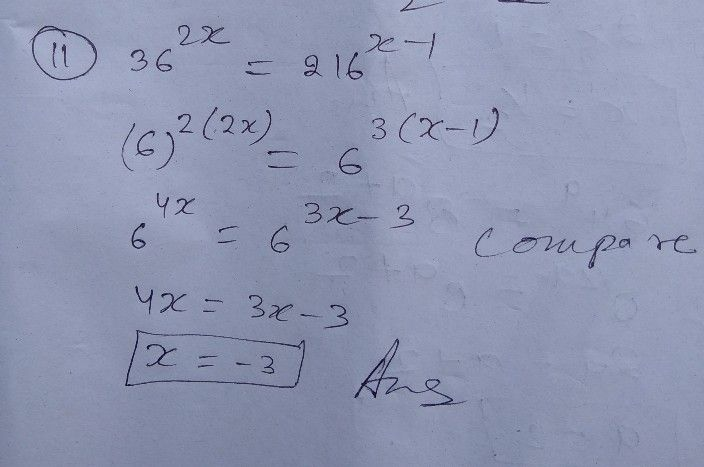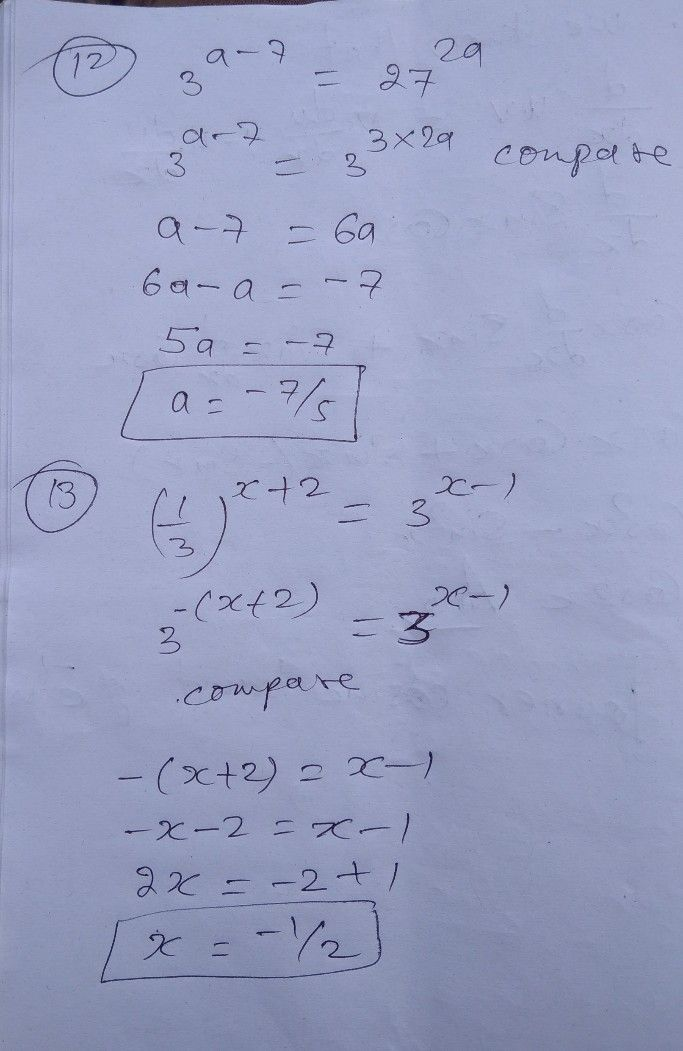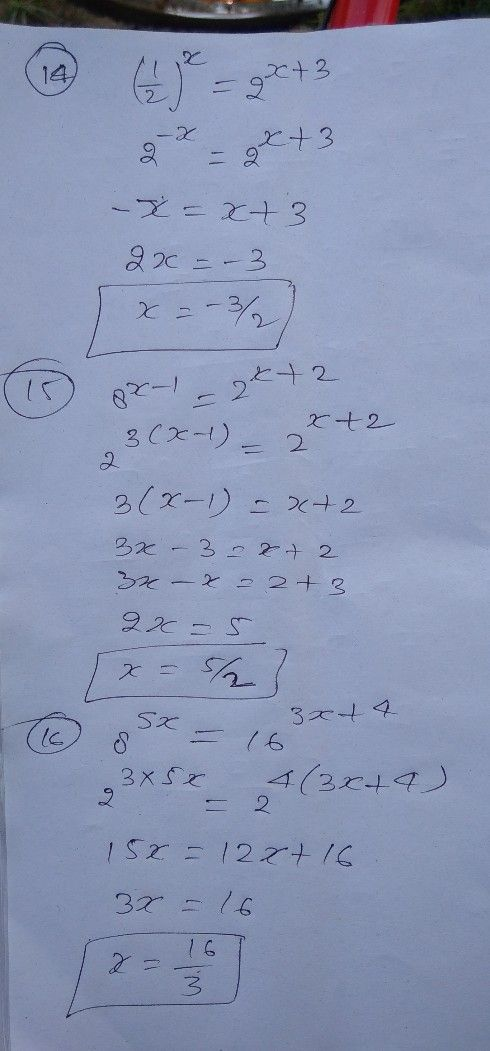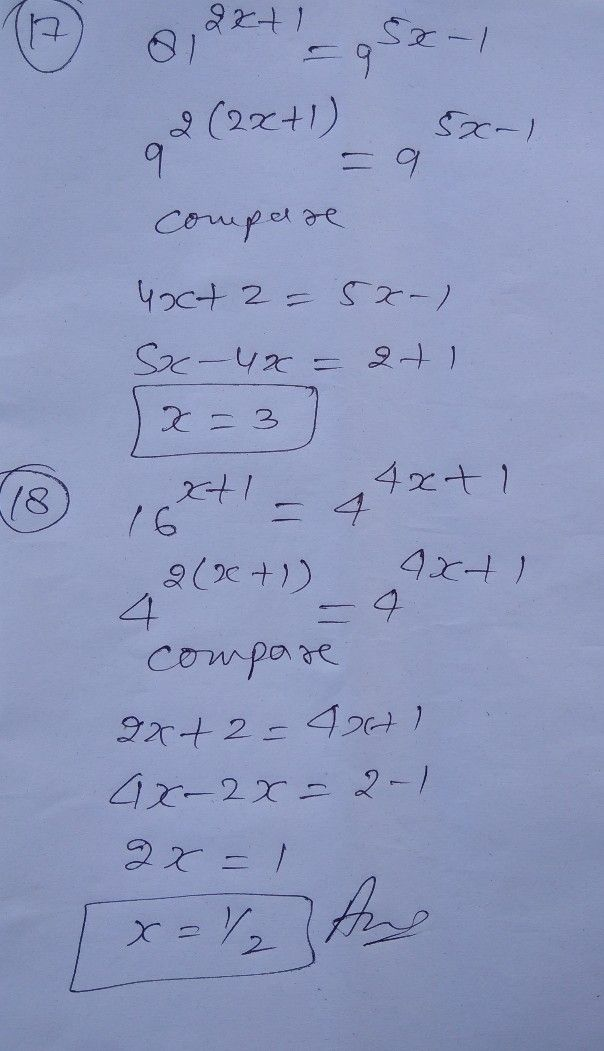Symbol
Problem$11$ $36^{2x}=216^{x-1}$ $12$ $3a-7=27^{2a}$ $13.\left(\dfrac {1} {3}\right)^{x+2}$ $=3x-1$ $14\left(\dfrac {1} {2}\right)^{x}$ $=2^{x43}$ $\bar{158^{x-1}=2} x+2$ $16^{-}$ $8$ $5x$ $=$ $16$ $3x+4$ $\bar{17}$ $81^{2}\bar{x+1}$ $=-$ $95x$ $=$ $1$ $1B$ $\bar{16x+1=4^{4x+}} 1$
10th-13th grade
Algebra
Search count: 110
Question content
Alg2+precal. Exponential equation
SolutionQanda teacher - NirmalyaStudent
14,15,16,17,18?Qanda teacher - NirmalyaStudent
Thanks